# ISNA Function In ExcelArticle byExcelMojo Team## What Is ISNA Function In Excel?

The ISNA function in Excel checks the cell values and states if the value is an “#N/A” error or not. The function returns TRUE if the specified value is #N/A error and FALSE otherwise.

The ISNA Excel function is an inbuilt function, so we can insert the formula from the “Functions Library” or enter it directly in the worksheet. For example, the below table contains a list of values in column A to check for #N/A error.

Select cell B2, enter the formula =ISNA(A2), and press the “Enter” key. Then, drag the formula from cell B2 to B10 using the fill handle. The output is shown below. Column C is for our reference.

[Output Observation: The result is TRUE only in the target cell B4, as the source data in cell A4 is the #N/A error. The ISNA() output is FALSE in the corresponding target cells in column B, as no other cell in column A contains the #N/A error.]

###### Key Takeaways
• The ISNA function in Excel checks if the given value is a #N/A error. And if the specified value is #N/A error, the function returns TRUE, else FALSE.
• We can use the ISNA() with the IF() and MATCH() to return a customized output instead of the #N/A error or the default logical results TRUE or FALSE.
• We can also use ISNA() with other Excel functions such as FIND() and SUMPRODUCT().
• When we directly enter the #N/A error as the argument, i.e., ISNA(#N/A), the output will be TRUE. However, if we provide the value argument as #N/A within double quotations (“#N/A”), the ISNA function in Excel will return the logical value FALSE as the output.

### ISNA() Excel Formula

The syntax of the ISNA Excel formula is:

The argument of the ISNA Excel formula is:

• value: The value or expression required to test for the #N/A error. It is a mandatory argument.

### How To Use ISNA Excel Function?

We can use the ISNA Excel Function in 2 methods, namely,

1. Access from the Excel ribbon.
2. Enter in the worksheet manually.

#### Method #1 – Access from the Excel ribbon

First, choose an empty cell for the output  → select the “Formulas” tab → go to the “Functions Library” group → click the “More Functions” option drop-down → click the “Information” option right arrow → select the “ISNA” function, as shown below.

The “Function Arguments” window pops up. Enter the argument value in the “Value” field → click “OK”, as shown below.

#### Method #2 – Enter in the worksheet manually

1. Select an empty cell for the output.
2. Type =ISNA( in the cell. [Alternatively, type =IS and select the ISNA function for the Excel suggestions.]
3. Enter the argument as cell values or cell references in Excel and close the brackets.
4. Finally, press the “Enter” key to view the result.

Let us take an example. Here, we will check if each value is a #N/A error or not using ISNA function and the IF() Excel function.

The following table contains different data values in column A.

The steps to find the #N/A error using ISNA function in Excel and the IF() function are:

1. Select cell B2, enter the formula =IF(ISNA(A2), “The value is #N/A error”,A2), and press the “Enter” key.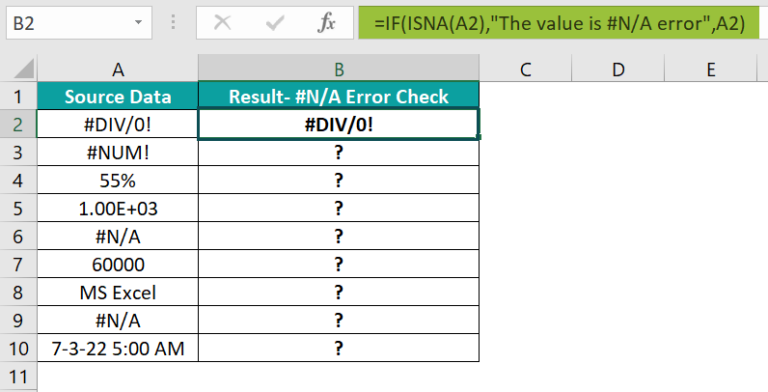2. Drag the formula from cell B2 to B10 using the fill handle.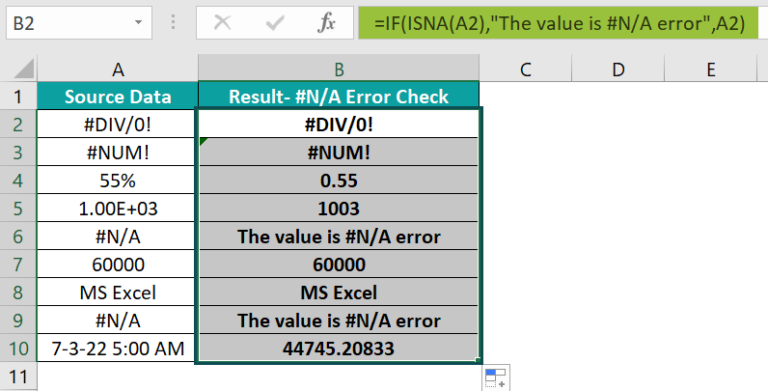We get the output as shown above.

[Output Observation: Cells A6 and A9 have the #N/A error, so the corresponding cells B6 and B9 return the conditional result, and all the other cells return the same value according to the IF() condition.]

### Examples

We will understand some advanced scenarios with the ISNA function in Excel examples.

#### Example #1

We will use the ISNA with FIND function in Excel to display the phrases’ positions.

The following table contains text values in column A and the phrase to find in column B.

The steps to find the #N/A error using the ISNA function in Excel with FIND() functions are:

• Step 1: Select cell C2, enter the formula =ISNA(FIND(B2,A2)), and press the “Enter” key.

[In the above formula, the FIND() first attempts to find the letter “l” in the cell A2 text value. And as the given text in cell A2 contains the first instance of the required letter at the 13th position, the function returns the value 13. And so, the input for the ISNA function in Excel becomes 13, thus making the function return the logical value FALSE.

• Step 2: Drag the formula from cell C2 to C6 using the fill handle.

We get the output as shown above.

[Output Observation: In the case of the target cell C6 formula, the given text is the #N/A error. So, the FIND() output is the #N/A error. And thus, as the input for the ISNA function in Excel is the #N/A error, the final output is TRUE.

#### Example #2

We will use the ISNA with IF() and MATCH() function in Excel to compare two columns for matches and differences.

The below table shows a list of employees with car parking slots mapped to their employee IDs. And column C contains a list of employee IDs.

The steps to use the ISNA function with IF() and MATCH() to get the required data are:

• Step 1: Select cell D2, enter the formula =IF(ISNA(MATCH(C2,\$A\$2:\$A\$11,0)),”Employee Does Not Have A Car Parking Slot”,”Employee Has A Car Parking Slot”), and press the “Enter” key.
• Step 2: Drag the formula from cell D2 to D11 using the fill handle.

We get the output as shown above.

[Output Observation: Let us consider the target cell D11 formula. First, the MATCH() checks the relative position of cell C11 value, ATL98459, in column A. And as the employee ID is not present in column A, the MATCH() returns the #N/A error. Then, the ISNA(#N/A) returns TRUE, and as the IF condition hold, the IF() returns the phrase “Employee Does Not Have A Car Parking Slot” as output.]

#### Example #3

We will use the ISNA with MATCH and SUMPRODUCT function in Excel to count the number of #N/A errors in a given cell range.

The following image shows two tables. The first table contains the full list of grocery items and the second table contains a list of items to refill in the pantry.

The procedure to use the ISNA function in Excel with SUMPRODUCT() and MATCH() is:

• Select cell B13, enter the formula =SUMPRODUCT(–ISNA(MATCH(A2:A11,C2:C6, 0))), and press the “Enter” key.

We get output as 5, as shown below.

[Output Observation: In the above formula, the MATCH() first returns the relative position of each grocery item available in column A, according to its placement in column C. So the MATCH() output is {3;#N/A;2;#N/A;1;#N/A;#N/A;4;5;#N/A}. Please note that the function returns the position as #N/A for items available in column A but not in column C.

Thus, the ISNA() output is {FALSE;TRUE;FALSE;TRUE;FALSE;TRUE;TRUE;FALSE;FALSE;TRUE} and the double negation () enforces the logical values into ones and zeros. And finally, the SUMPRODUCT() adds them up to return the value, 5.

### Important Things to Note

• To provide the #N/A error directly as an argument to the ISNA() function, we must enter it in the function without double quotes to get the logical value TRUE as the output.
• The ISNA function in Excel’s argument can be a cell address of the value or an expression evaluating the value we require to check for the #N/A error.
• We must ensure to type the exact function name as there are multiple IS() group functions. If incorrectly entered, we will get incorrect results or the “#Name?” error.

1. Where is the ISNA function in Excel?

The ISNA function in Excel is in the FormulasMore FunctionsInformationISNA to apply the function in the specific target cell.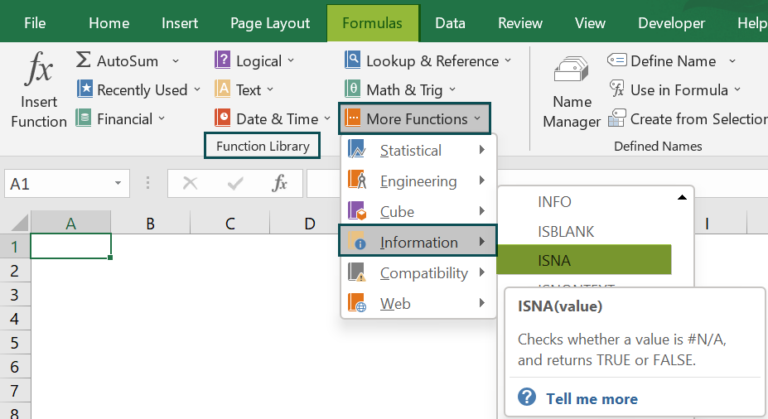2. How to use the ISNA function with VLOOKUP in Excel?

We can use the ISNA function with VLOOKUP in Excel by applying the following formula.
=IF(ISNA(VLOOKUP(….),”customized_text”,VLOOKUP(….))

For example, the below image shows two tables. The first table contains a list of office supplies and their price details, and the second table shows a list of required items.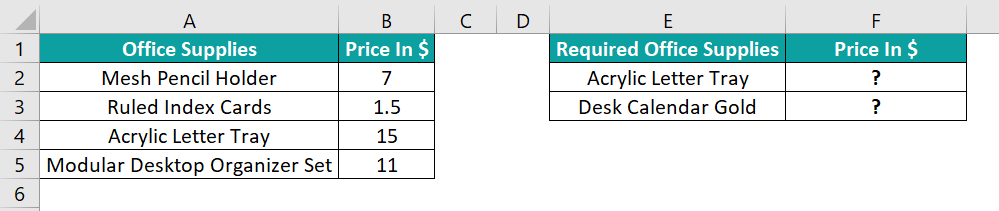The steps to use the ISNA function with VLOOKUP are:

• Step 1: Select cell F2, enter the formula, =IF(ISNA(VLOOKUP(E2,\$A\$1:\$B\$5,2,0)),”Data Not Available”,VLOOKUP(E2,\$A\$1:\$B\$5,2,0)), and press the “Enter” key.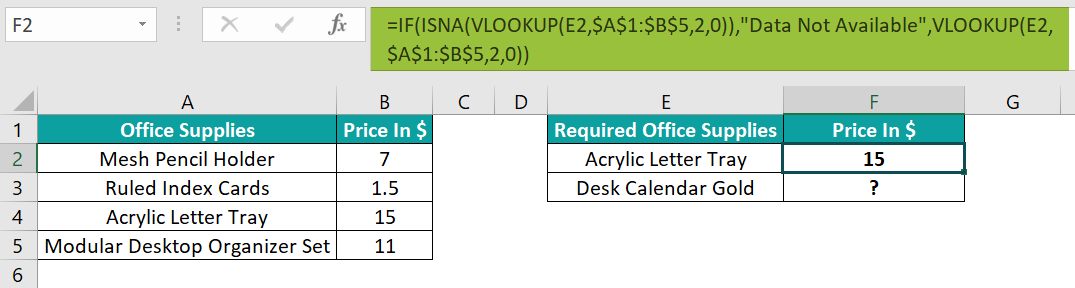• Step 2: Drag the formula from cell F2 to F3 using the fill handle.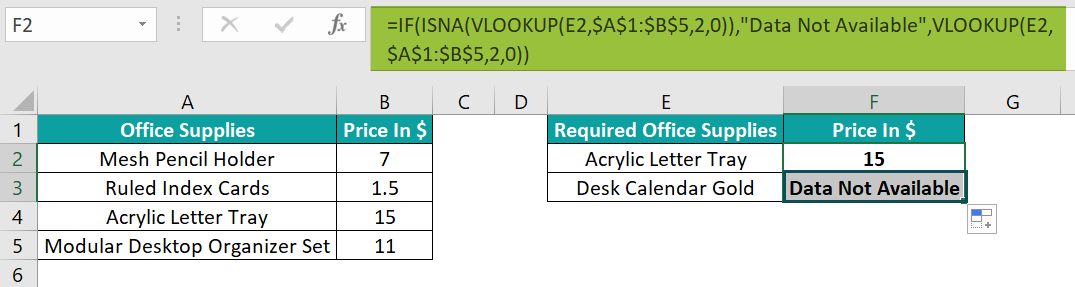The output is shown above.

[Output Observation: Let us consider the formula’s working in target cell F3. First, the VLOOKUP() inside the ISNA() looks up the price of the cell E3 value, Desk Calendar Gold, in column B of the first table. And as the specific item’s data is not available in the first table, the VLOOKUP() returns the #N/A error. So, the ISNA(#N/A) returns the logical value TRUE as the output. Thus, the IF condition holds. And hence, it returns the phrase “Data Not Available” as the final output.]

3. Why is the ISNA function in Excel not working?

The ISNA function in Excel may not work for the following reasons:
If the supplied argument is #N/A error within double quotations.
The function is incorrectly entered.
If the argument is an expression that does not evaluate the value to test for the #N/A error.